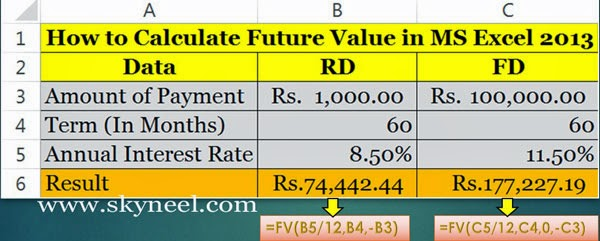# How to Calculating Future Value in Excel

The Calculating Future Value in Excel is a financial function, An investment worth after a time with constant interest rate and payment.

If anybody want to invest his money in future investment then first he want to know what the return of the investment is after completed the specific time. So, it is very important to know how to calculating future value in MS Excel? FV() is the most popular financial function of Microsoft Excel. This function is used to returns future value of investment which is based on a specific periodic, fixed payment and fixed rate of interest.

### Syntax of FV:

FV(rate, pmt, nper, pv, type) rate: Annual Interest Ratenper: Number of Periodpmt is the payment monthly installment which is made on each period. It can be feed as a negative number.pv: Present/Principal Value   type: It’s an optional value. If you feed 0, it means end of the period and if you feed 1, If you ignore this part then it is assumed to be 0.

Example:When you want to calculate future value of an investment like invest amount Rs. 1000/- for 60 months @ 8.50% annual interest rate in the case of RD (Recurring Deposit) If you want to calculate future value of an investment like investment amount Rs. 1,00,000/- for 60 months @ 11.50% annual interest rate in the case of FD (Fixed Deposit) then, you have to take following steps:

## Easily Calculating Future Value in Excel

Step 1: Prepare the following sheet in MS Excel.Step 2: If you want to calculate RD (Recurring Deposit) amount, then you have to type following formula in Cell B6  =FV(B5/12,B4,-B3) then press enter it will show you Rs. 74,442.44/- as a result.

Step 3: If you want to calculate FD (Fixed Deposit) amount then you have to type following formula in Cell C6 =FV(C5/12,C4,0,-C3) then press enter it will show you Rs. 1,77,227.19/- as a result.I hope you like this post and also sort out your problem regarding to calculating future value in excel. Share this post in your friends and improve your knowledge. Give your important suggestions in the comment box. Thanks.